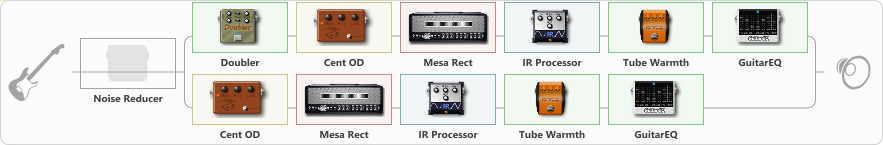# Double Mesa

Discussion in 'ToneLib-GFX presets' started by Blbulator, Aug 28, 2023.

1. Double Mesa

Preset name: MESA GOOD

Effects chain:Effect: "Noise Reducer" (Dynamics / Filter), active - "no"
{
"Sens" = 81
"Mode" = Soft
}

Effect: "Splitter" (Dynamics / Filter)
{
"A-Bypass" = Off
"A-Pan" = -70
"A-Level" = 55
"B-Bypass" = Off
"B-Pan" = 70
"B-Level" = 55
"Width" = 100

'A' branch:
{

Effect: "Doubler" (Dynamics / Filter), active - "yes"
{
"A-Dly" = 60
"A-Inv" = Off
"A-Level" = 61
"B-Dly" = 64
"B-Inv" = Off
"B-Level" = 61
}

Effect: "Cent OD" (Overdrive / Distortion), active - "yes"
{
"Drive" = 10
"Tone" = 50
"Level" = 100
}

Effect: "Mesa Rect" (Amp simulators), active - "yes"
{
"Gain" = 29
"Bass" = 50
"Middle" = 40
"Treble" = 60
"Presence" = 63
"Master" = 60
"Level (dB)" = 3
}

Effect: "IR Processor" (Cabinets), active - "yes"
{
"IR" = TM_RAW_03-Marv30
"Low Cut (Hz)" = 109
"Hi Cut (kHz)" = 6.0
"Mix" = 100
"Level (dB)" = 0
}

Effect: "Tube Warmth" (Dynamics / Filter), active - "yes"
{
"Input" = 55
"Warmth" = 75
"Level" = 75
}

Effect: "GuitarEQ" (Dynamics / Filter), active - "yes"
{
"160 Hz" = 0
"400 Hz" = 0
"800 Hz" = -2
"1.6 kHz" = 7
"3.2 kHz" = 1
"6.4 kHz" = 0
"12 kHz" = 0
"Level (dB)" = 0
}
}
'B' branch:
{

Effect: "Cent OD" (Overdrive / Distortion), active - "yes"
{
"Drive" = 10
"Tone" = 50
"Level" = 100
}

Effect: "Mesa Rect" (Amp simulators), active - "yes"
{
"Gain" = 30
"Bass" = 50
"Middle" = 40
"Treble" = 60
"Presence" = 64
"Master" = 60
"Level (dB)" = 3
}

Effect: "IR Processor" (Cabinets), active - "yes"
{
"IR" = TM_RAW_03-Marv30
"Low Cut (Hz)" = 103
"Hi Cut (kHz)" = 6.0
"Mix" = 100
"Level (dB)" = 0
}

Effect: "Tube Warmth" (Dynamics / Filter), active - "yes"
{
"Input" = 55
"Warmth" = 76
"Level" = 75
}

Effect: "GuitarEQ" (Dynamics / Filter), active - "yes"
{
"160 Hz" = 0
"400 Hz" = 0
"800 Hz" = -2
"1.6 kHz" = 7
"3.2 kHz" = 1
"6.4 kHz" = 0
"12 kHz" = 0
"Level (dB)" = 0
}
}
}

Note: You will need to download and install the ToneLib-GFX software to use the preset.

#### Attached Files:

• ###### Double_Mesa.tlgfx
File size:
31.9 KB
Views:
735
pit72 and M Kingshott like this.